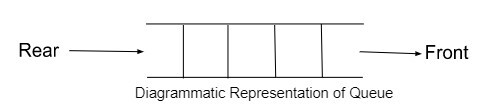# Basic Operations for Queue in Data Structure

Queue is a collection of different data types and is an important part of data structure, following a particular order to insert and remove elements. In this tutorial, we will understand the basic operations of the queue.

## What is Queue in Data Structure?

A Queue is a linear data structure that resembles a queue in real life. You all have been part of some queue in school, the billing counter, or any other place, where the one entered first will exit first in the queue. Similarly, a queue in data structure also follows the FIFO principle, which defines First In First Out. The element inserted first in the queue will terminate first, compared to the remaining.

A queue has two endpoints and is open to both ends.

• Front − It is the end from where elements move out of the queue.

• Rear − It is the end from where elements are inserted in the queue.It can be implemented using a one-dimensional array, pointer, structures, and linked list. C++ library contains various built-in functions that help to manage the queue, its operations take place only at the front and rear ends.

## Syntax to declare a queue

queue<data type> queue_name


## Example

queue<int> q
queue<string> s


## Basic Queue Operations

The most useful operation of the queue in C++ are as follows −

• pop() −It removes the front element of the queue. Syntax −queue_name.pop();

• push() −(): It is used to insert elements at the starting or rear end of the queue. Syntax −queue_name.push(data_value);

• front() −(): It checks or returns the element at the front of the queue. Syntax −queue_name.front();

• size() −It is used to get the size of the queue. Syntax −queue_name.size();

• empty() −It checks if the queue is empty or not. Returns boolean value as per the condition. Syntax −queue_name.empty();

## Code for the push() function.

#include <iostream>
#include<queue>

using namespace std;

int main() {
queue<int> q; //initializing queue
q.push(4); //inserting elements into the queue using push() method
q.push(5);
q.push(1);
cout<<"Elements of the Queue are: ";

while(!q.empty()) {
cout<<q.front()<<"";  // printing 1st element of the queue
q.pop();  // removing elements from the queue
}
return 0;
}


## Output

Elements of the queue are: 451


In the above example, we created a queue q and inserted elements into it using the push() function, the function inserts all elements at the rear end.

Use the empty() function to check if the queue is empty or not, if not, the queue will return the element at the front, and using the pop() function will remove queue elements from the front end.

## Example

#include <iostream>
#include<queue>

using namespace std;

int main() {
queue<int> q; //initializing queue
q.push(4); //inserting elements into the queue using push() method
q.push(5);
q.push(1);
cout<<"Elements of the Queue are: ";

while(!q.empty()) {
cout<<q.front()<<""; // printing 1st element of the queue
q.pop(); // removing elements from the queue
}
return 0;
}


## Output

size of queue is : 451


## Example of empty() function of the queue.

#include <iostream>
#include<queue>

using namespace std;

int main() {
queue<string> q; //declaring string type of queue
q.push("cpp"); //inserting elements into the queue using push() method
q.push("Java");
q.push("C++");

if(q.empty()) //using empty() function to return the condition
cout<<"yes, Queue is empty";
else
cout<<"No, queue has elements";

return 0;
}


## Output

No queue has elements


## Conclusion

A queue can store integer and string elements. In the data structure, there is one more queue called the priority queue, which has priority with all the queue elements.

I hope this tutorial will help you to understand the meaning of queue in the data structure.